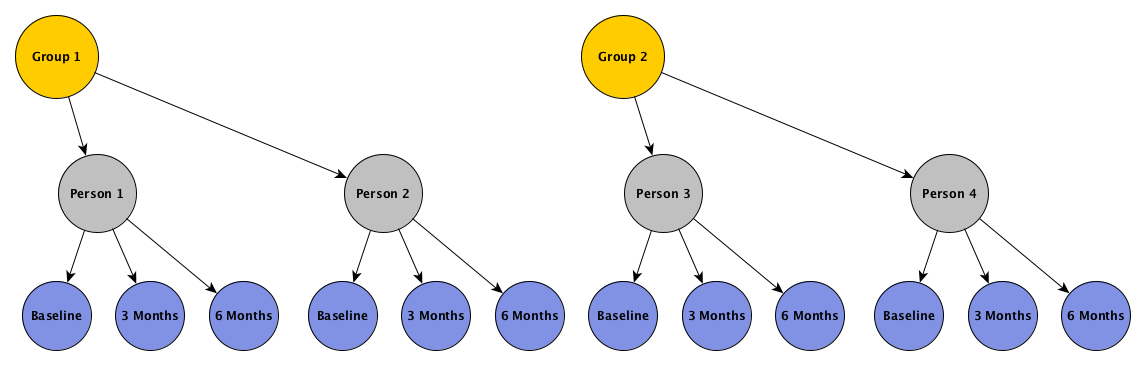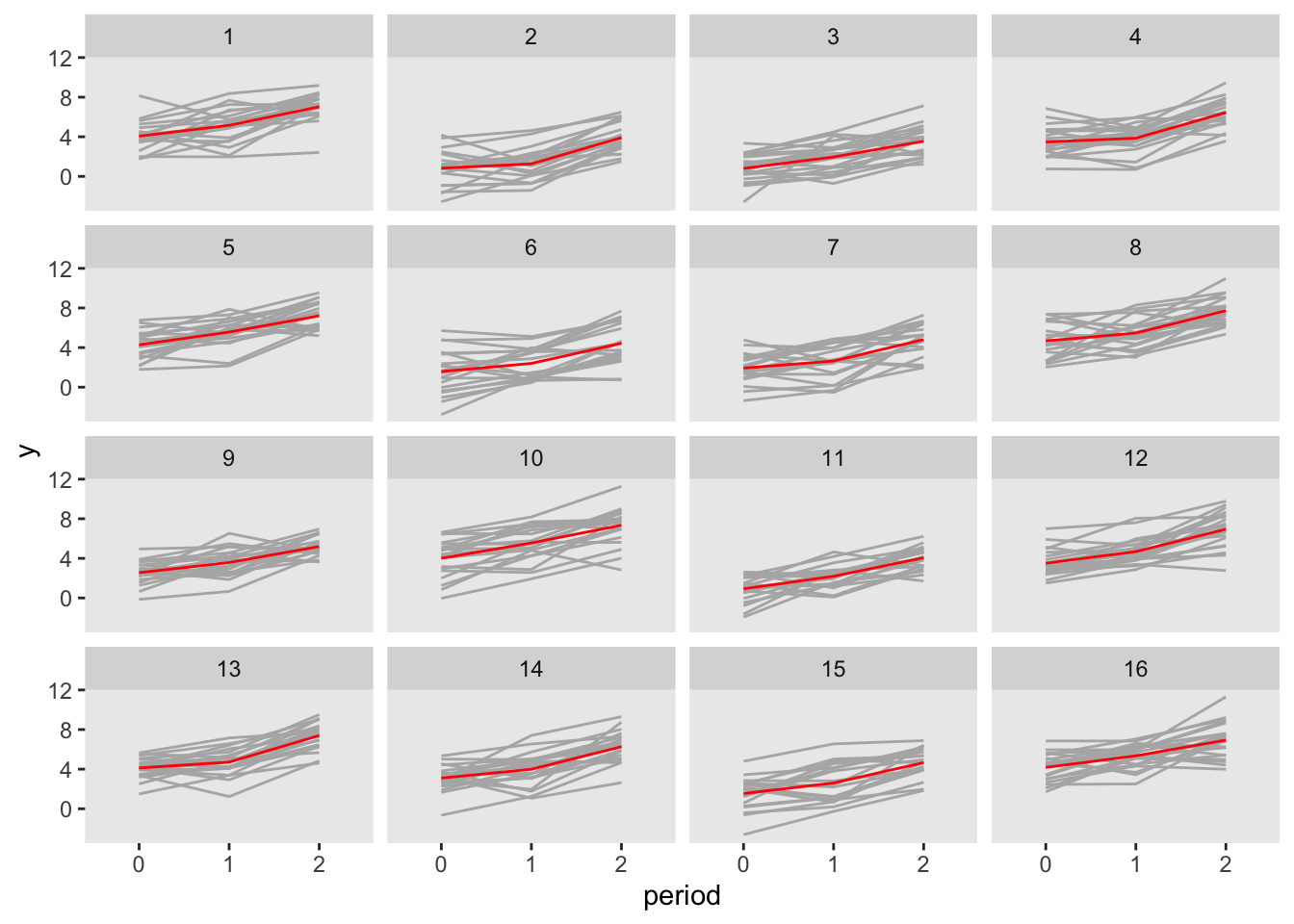# There's always at least two ways to do the same thing: an example generating 3-level hierarchical data using simstudy

“I am working on a simulation study that requires me to generate data for individuals within clusters, but each individual will have repeated measures (say baseline and two follow-ups). I’m new to simstudy and have been going through the examples in R this afternoon, but I wondered if this was possible in the package, and if so whether you could offer any tips to get me started with how I would do this?”

This question popped up in my in-box a couple of days ago. And since I always like an excuse to do a little coding early in the morning to get my brain going, I decided to create a little example, though in this case, there were at least two ways to go about it. I sent back both options, and am putting them up here, since I know this kind of data generation problem comes up frequently. In fact, the post I recently wrote on open cohorts in stepped-wedge designs had to deal with this same issue, though in a slightly more elaborate way.

### Three-level hierarchical data

In this example, we want individuals clustered within groups, and measurements clustered within individual, as depicted by this figure:The hierarchical scheme represented implies that outcomes for individuals within groups are correlated, and that measurements over time for a particular individual are correlated. The structure of these two levels of correlation can take on a variety of forms. In the examples that follow, I am going to assume that the correlation between the individuals in a group is constant, as are the individual measurements over time. We could easily make the assumption that measurements closer in time will be more highly correlated than measurements further apart in time (such as auto-regressive correlation with 1 period of lag), but since we have only three measurements, it is not totally unreasonable to assume constant correlation.

### Generating data explicitly with random effects

Enough with the preliminaries - let’s get to the data generation. In the first approach, both levels of correlation will be induced with group- and individual-level random effects using the following underlying model:

$Y_{ijt} = \beta_t + \gamma_j + \alpha_i + \epsilon_{ijt},$

where $$Y_{ijt}$$ is the outcome for person $$i$$ in group $$j$$ during time period $$t$$. $$\beta_t$$ is the mean outcome during period $$t$$, $$t \in \{ 0,3, 6 \}$$. $$\gamma_j$$ is the group-specific effect, and $$\gamma_j \sim N(0,\sigma^2_\gamma)$$. $$\alpha_i$$ is the individual-specific effect, and $$\alpha_i \sim N(0,\sigma^2_\alpha)$$. Finally, $$\epsilon_{ijt}$$ is the noise for each particular measurement, where $$\epsilon_{ijt} \sim N(0,\sigma^2_\epsilon)$$.

The group, individual, and outcome definitions are the first order of business. In this example, $$\sigma^2_\gamma = 2$$, $$\sigma^2_\alpha = 1.3$$, and $$\sigma^2_\epsilon = 1.1$$. In addition, the average outcomes at baseline, 3 months and 6 months, are 3, 4, and 6, respectively:

library(simstudy)

### Group defintion

defg <- defData(varname = "gamma", formula=0, variance = 2, id = "cid")

### Individal definition

defi <- defDataAdd(varname = "alpha", formula = 0, variance = 1.3)

### Outcome definition

defC <- defCondition(condition = "period == 0",
formula = "3 + gamma + alpha",
dist = "nonrandom")
defC <- defCondition(defC, condition = "period == 1",
formula = "4 + gamma + alpha",
dist = "nonrandom")
defC <- defCondition(defC, condition = "period == 2",
formula = "6 + gamma + alpha",
dist = "nonrandom")

defy <- defDataAdd(varname = "y", formula = "mu", variance = 1.1)

To generate the data, first we create the group level records, then the individual level records, and finally the repeated measurements for each individual:

set.seed(3483)
dgrp1 <- genData(100, defg)

dind1 <- genCluster(dgrp1, "cid", numIndsVar = 20, level1ID = "id")

dper1 <- addPeriods(dind1, nPeriods = 3, idvars = "id")
dper1 <- addCondition(defC, dper1, newvar = "mu")

dper1 <- addColumns(defy, dper1)

Here is a plot of the outcome data by period, with the grey lines representing individuals, and the red lines representing the group averages:Here is a calculation of the observed covariance matrix. The total variance for each outcome should be close to $$\sigma^2_\gamma + \sigma^2_\alpha +\sigma^2_\epsilon = 4.4$$, and the observed covariance should be close to $$\sigma^2_\gamma + \sigma^2_\alpha = 3.3$$

dcor1 <- dcast(dper1, id + cid ~ period, value.var = "y")
setnames(dcor1, c("id", "cid", "y0", "y1", "y2"))

dcor1[, cov(cbind(y0, y1, y2))]
##     y0  y1  y2
## y0 4.5 3.2 3.4
## y1 3.2 4.2 3.2
## y2 3.4 3.2 4.6

The correlation $$\rho$$ show be close to

$\rho = \frac{\sigma^2_\gamma + \sigma^2_\alpha}{\sigma^2_\gamma + \sigma^2_\alpha +\sigma^2_\epsilon} = \frac{3.3}{4.4} = 0.75$

(For a more elaborate derivation of correlation coefficients, see this post on stepped-wedge designs.)

dcor1[, cor(cbind(y0, y1, y2))]
##      y0   y1   y2
## y0 1.00 0.73 0.75
## y1 0.73 1.00 0.73
## y2 0.75 0.73 1.00

### Directly generating correlated data

In this second approach, the group-level correlation is once again generated using a group effect. However, the individual-level effect is replaced by noise that is explicitly correlated across time. The model here is

$Y_{ijt} = \beta_t + \gamma_j + \phi_{ijt},$

where the noise $$\mathbf{\phi}_{ij}$$ is a vector of noise components $$\{\phi_{ij0},\phi_{ij3},\phi_{ij6}\} \sim N(\mathbf{0}, \Sigma)$$, and

$\Sigma = \left [ \begin{matrix} \sigma^2_\phi & \rho \sigma^2_\phi & \rho \sigma^2_\phi \\ \rho \sigma^2_\phi & \sigma^2_\phi & \rho \sigma^2_\phi \\ \rho \sigma^2_\phi & \rho \sigma^2_\phi & \sigma^2_\phi \end{matrix} \right ]$

In this case $$\sigma^2_\gamma$$ is still 2, and $$\sigma^2_\phi = 2.4$$ to ensure that total variation is 4.4. We set $$\rho = 0.54167$$ so that the $$\rho \sigma^2_\phi = 1.3$$, ensuring that the overall covariance of the observed outcome $$y$$ across periods is $$3.3$$ as in the first method.

defg <- defData(varname = "gamma",
formula = 0, variance = 2, id = "cid")
defg <- defData(defg, varname = "mu",
formula = 0, dist = "nonrandom")
defg <- defData(defg, varname = "phi",
formula = 2.4, dist = "nonrandom")

defC <- defCondition(condition = "period == 0",
formula = "3 + gamma + e",
dist = "nonrandom")
defC <- defCondition(defC, condition = "period == 1",
formula = "4 + gamma + e",
dist = "nonrandom")
defC <- defCondition(defC, condition = "period == 2",
formula = "6 + gamma + e",
dist = "nonrandom")

In the data generation process, the function addCorGen is used to create the correlated noise across time:

set.seed(3483)
dgrp2 <- genData(100, defg)

dind2 <- genCluster(dgrp2, "cid", numIndsVar = 20, level1ID = "id")

dper2 <- addPeriods(dind2, nPeriods = 3, idvars = "id")
dper2 <- addCorGen(dper2, "id", nvars = 3, param1 = "mu", param2 = "phi",
rho = .54167, dist = "normal", corstr = "cs", cnames = "e")
dper2 <- addCondition(defC, dper2, newvar = "y")

I won’t do a second plot, because it would look identical to the one above. But I am calculating the covariance and correlation matrices for the outcome to illustrate for you that the two slightly different approaches do indeed generate similarly distributed data.

dcor2 <- dcast(dper2, id + cid ~ period, value.var = "y")
setnames(dcor2, c("id", "cid", "y0", "y1", "y2"))

dcor2[, cov(cbind(y0, y1, y2))]
##     y0  y1  y2
## y0 4.4 3.4 3.3
## y1 3.4 4.4 3.4
## y2 3.3 3.4 4.5
dcor2[, cor(cbind(y0, y1, y2))]
##      y0   y1   y2
## y0 1.00 0.76 0.75
## y1 0.76 1.00 0.76
## y2 0.75 0.76 1.00

In the example here, I wouldn’t say either approach is better. For some, the purely random effects approach may be more intuitive, and for others the correlated noise might be. However, if we want a more complex correlation pattern, like the AR-1 pattern I mentioned earlier, one approach may in fact be a little more straightforward to implement.

And no, I don’t respond so thoroughly to every question I get; sometimes it is better for you to struggle a bit to figure something out.